TigerJython4Kids micro:bit Robotik
crashcourse
 Deutsch   English   Français

## 1. PYTHON CRASH COURSE### YOU LEARN HERE...

 the most important programming concepts in Python by studying elementary examples with a simple model of a glowbug (firefly), which you can move on the LED display with forward(), left(), right() and back() commands. If you already worked through the chapter Turtle Graphics or if you already acquired equivalent basic Python knowledge, you may skip this chapter and go straight to the next.### SEQUENCE

A computer program consists of a sequence of program lines, which are processed one after the other. In order to use the commands for the beetle, you have to import the module mbutils with from mbglow import *.

 When this import line is processed, a beetle is created. It is shown as a lit LED and when the beetle moves, the visited positions remain turned on, so the beetle leaves a trackl. Your commands will be executed after downloading the program to the micro:bit. All commands are written in English and always end with a parameter bracket. This can contain further information about the command. For every word in the program, the capitalization must be kept exactly.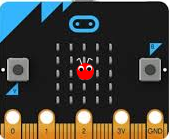With forward() the beetle moves one step forward in the current direction of movement. At program start, the direction of movement points upwards. left(90) turns the beetle 90 degrees to the left and right(90) 90 degrees to the right. But this will not be noticeable until the next forward() command.

With the following program, the beetle draws the track shown:

 Program: ```from mbglow import * makeGlow() forward() left(90) forward() right(90) forward() ``` from mbglow import * makeGlow() forward() left(90) forward() right(90) forward() ► Copy to clipboard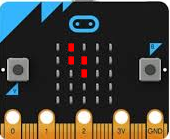You can type the program in the editor window or copy/paste it from the template. To do this, click on Copy to clipboard and insert it with Ctrl+V into the TigerJython editor.

 To download the program to the micro:bit and execute it there, click on the black button in the task bar (Download/Execute).### REPEAT LOOP

 To traverse a square, the beetle must execute the commands forward() and left (90) four times. You save paperwork and simplify the code considerably if you use a repeat loop. You just write repeat 4: (the colon is important). The commands that are to e repeated must all be equally indented. You use four spaces or the tab key.Program:

```from mbglow import *

makeGlow()

repeat 4:
forward()
left(90)
```
► Copy to clipboard

 With setPos(x, y) the beetle jumps to a new position, where x and y are in the range -2, -1, 0, 1, or 2 (check the x-y coordinate system in the figure). In the next program you want to draw the largest possible square. To do so, first set the beetle to the lower left corner. To draw a square side, move the beetle 4 times forward by using a repeat loop. This will be a nested (inner) loop inside the outer repeat loop.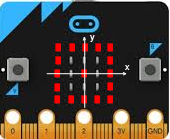Pay attention to the correct indentation!

Program:

```from mbglow import *

makeGlow()

setPos(-2, -2)
repeat 4:
repeat 4:
forward()
right(90)
```
► Copy to clipboard

### FUNCTIONS (NAMED PROGRAM BLOCKS)

It is a good practice to group together commands that perform a common action. In Python such a program block is called a function, and you reference it by its name. After the function definition, the function may be used like an additional command: Each time you want the program block to execute, you just write the function name. You say that you invoke or call the function. The new function is available everywhere in your program. It's like extending  the vocabulary of the Python language.

The use of functions is very important, because you structure the program by breaking it into smaller pieces that have individual purposes. Each time you need to perform these actions, you do not duplicate the program lines, but just call the function.

 In your example, you define a function square() that moves the beetle on a square. The function definition starts with the keyword def. Then follow the function name, a parameter bracket and a colon. The lines in the function body must be indented. The function is executed when the function is called. The function definitions are always in the upper part of the program.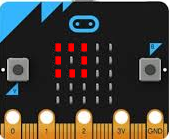As a function name, choose an identifier that expresses something about what the function does. You may not use special characters (spaces, umlauts, accents, punctuation, etc.). As a convention always start the name with a lowercase letter.

Program:

```from mbglow import *

makeGlow()

def square():
repeat 4:
forward()
forward()
left(90)

square()
```
► Copy to clipboard

 You can call a function several times. To create the adjacent image, call the square() function 4 times. With the command setSpeed(80) the beetle runs faster. (The default value is 50 and values in the range 0 to 100 are allowed.)Program:

```from mbglow import *

makeGlow()

def square():
repeat 4:
forward()
forward()
left(90)

setSpeed(80)
repeat 4:
square()
right(90)
```
► Copy to clipboard

### VARIABLES

 In computer science, variables are placeholders for values that change in the course of program execution. So a variable has a name and a value. In the next program you use the variable v, which stands for the speed of the beetle, which changes in the course of the program. At the beginning, you set the value to 30 using the assignment operation v = 30. After each invocation of square(), v increases by 20.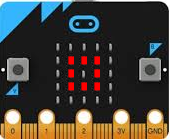To increase the value of v by 20, you make the assignment v = v + 20, which at first glance looks like an mathematical equation, but is something completely different: It tells the computer to take the current value of v and add 20 to it, and then save the result in v again.

The command showTrace(False) causes the beetle to leave no trace.

Program:

```from mbglow import *

makeGlow()

def square():
repeat 4:
forward()
forward()
right(90)

v = 30
showTrace(False)
setPos(-1, -1)

repeat 4:
setSpeed(v)
square()
v = v + 20
```
► Copy to clipboard

### WHILE LOOP

The while loop is one of the most important program structures. It can be used universally for any kind of repetition, and occurs in virtually all programming languages. A while loop is started with the keyword while followed by a condition and a colon. As long as the condition is fulfilled, the commands in the subsequent program block are repeated. Colloquially this would be expressed as follows:

As long as condition is true, perform...

To express the condition, the comparison operators < (less), <= (less-or-equal), > (greater), >= (greater or equal), == (equal), != (different)  are used. Note the duplication of the equality sign in the equality test to make the difference to an assignment.

 In your example, the beetle moves 2 steps forward, then 2 steps backwards, turns 45°, then moves 2 steps forward, etc., until it points north again. The total angle of rotation is stored in the variable a. You start with a = 0 and add 45 after each loop. As long as the condition a <= 360 is true, the instructions in the while block (called the body) are executed.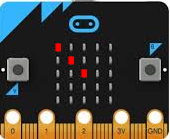Program:

```from mbglow import *

makeGlow()

setSpeed(90)
showTrace(False)
a = 0
while a <= 360:
forward()
forward()
back()
back()
left(45)
a = a + 45
```
► Copy to clipboard

 In robotics, a so-called "endless while loop" is often used. This is initiated with while True: Since True is always true, the loop will be executed endlessly. (Until a severe intervention: you download a new program, you click the reset button, you click CTRL+C in the console window or you remove the power from the micro:bit). clear() clears all LEDs.Program:

```from mbglow  import *

makeGlow()

def square():
repeat 4:
forward()
forward()
left(90)

clear()
setSpeed(90)
showTrace(False)
setPos(2, 0)
left(45)
while True:
square()
```
► Copy to clipboard

### FOR IN RANGE LOOP

 Often you need a whole-numbered variable in a repeat loop, which increases by one with each pass. You can solve this by using a counter variable with a while loop, but it's easier with a for loop, where the loop counter is automatically changed. for i in range (n) traverses numbers i from 0 to n-1 and for i in range (a, b) runs through numbers i from a to b-1. Note that the final value n or b is never included.With for x in range(-2, 3) x passes through the values -2, -1, 0, 1, 2. The LEDs in the top row are switched on one after the other. With sleep(500) you can pause the program execution for 500 milliseconds.

Program:

```from mbglow import *

makeGlow()

clear()
for x in range(-2, 3):
setPos(x, 2)
sleep(500)
```
► Copy to clipboard

 Often nested for- loops are used, for example, if you want to turn on all the LEDs one row at a time. First, the lowest row is switched on with y = -2, then with y = -1 the second lowest row, etc.Program:

```from mbglow  import *

makeGlow()

clear()
for y in range(-2, 3):
for x in range(-2, 3):
setPos(x, y)
sleep(300)
```
► Copy to clipboard

### SELECTION IF - ELSE

A selection is used when a program block should be executed only under a certain condition. The selection is initiated with the keyword if, followed by a condition and a colon. The following commands are part of the if block and are equally indented. They are executed only if the condition is true. The keyword else may follow, together with commands in an else block. These commands are executed, if the condition is false. In everyday language, one would express this as follows::

If condition true, then action1 else action2

In the following program the two buttons of the micro:bit are used: There is no else block.

 The beetle moves to the right. When it arrives at the right edge, it jumps back to the left edge and repeats this movement endlessly from left to right. So you move the beetle to the right with forward () and check its x-coordinate after each step. If the coordinate is 3, the beetle jumps back to the left edge (x = -2).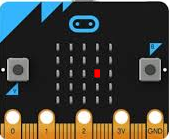Program:

```from mbglow import *

makeGlow()

right(90)
x = 0
showTrace(False)

while True:
forward()
x = x + 1
if x == 3:
setPos(-2, 0)
x = -2
```
► Copy to clipboard

In the next program, the beetle will randomly walk two steps forward or two steps backwards, then stop briefly, clear the track and return to the starting position. The action is repeated 10 times.

 Here you use random numbers. The function randint (a, b) is part of the module random and generates a number between a and b.(a and b are included, so randint(1, 6) generates all of the numbers 1,..,6). Therefore, the function randint(0, 1) randomly returns a 0 or a 1. If the value is 1, then the beetle advances 2 forward steps, otherwise (if the number is 0) 2 steps backward.Program:

```from mbglow import *
from random import randint

makeGlow()
setSpeed(80)
repeat 10:
r = randint(0, 1)
if r == 1:
forward()
forward()
else:
back()
back()
sleep(300)
clear()
setPos(0, 0)
```
► Copy to clipboard

### MEMO

 The basic program structures are sequence, repetition and selection. With a sequence, the commands are executed sequentially one after the other. With a repetition, program blocks are executed several times. Python uses the keywords while and for, in TigerJython the additional keyword repeat is available . The selection with the keywords if and else causes certain statements to be executed only under certain conditions. The else part can be omitted. Functions are important to structure the program. They avoid code duplication and serve to break up problems into smaller sub-problems. One speaks also of modularization.

### EXERCISES

1.
 Write a program using repeat, so that the beetle traces a plus sign.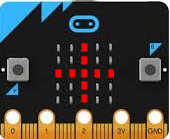2a.
 The beetle should draw a staircase with 4 steps.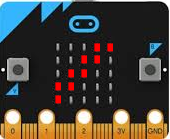2b.
 Solve the same task by defining a step() function that draws a single step.3.
 Write a program that turns on the LEDs in the two diagonals.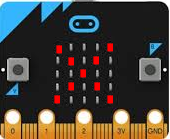4.
 Like in the first example of if-else, the beetle moves from left to right. But it should go through all the rows and turn on all LEDs. So the beetle starts in the lower left corner and moves to the right. When it reaches the right edge, it jumps back to the left edge and continues his movement in the second row.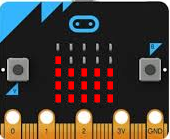5.
 Use two nested for loops to turn on all the LEDs on the display ```for y in range(-2, 3): for x in range (-2, 3): setPos(x, y) ``` Your program should run through all LEDs and turn on LEDs according to the adjacent figure.6.
Your program should turn on LEDs as in the "checkerboard" figure. Hint: The glowing LEDs meet the following condition: The sum of their x and y coordinates is an even number. For checking whether a number is even or odd, the modulo division a%b is used, which returns the remainder of the operation a divided by b.0-1
##### Didactic hints:
The keyword repeat does not belong to the syntax of Python, but was specifically built into TigerJython, so that repeat structures can be used before introducing the concept of variables. Repeat a number of times is perceived as very natural, because it is also used in everyday language.

If you want to remain compatible with Python, you can replace repeat n by for _ in range(n)
0-4
##### Didactic hints:

The concept of variables is fundamental, but a didactic challenge.

There are essentially three different methodological approaches. Since we only use variables in micro-bit robotics in simple contexts, we choose the pragmatic use.

 Low-Level-Modell Advantages Disadvantage A variable, as in the machine code / assembler, is considered a reserved main memory location, which is addressed via its address Clear reference to the machine structure Although addresses are important in assembler, C / C ++ and other programming languages with pointers, in practice they only play a minor role in many other programming languages or are even frowned upon

 Boxmetapher Advantages Disadvantage A variable is understood to be a box (or drawer) that is written with a name and in which the value is located A variable is understood to be a box (or drawer) that is written with a name and in which the value is located The box can also be empty, but a variable always has a value. The box can contain several things, but not a variable. The box metaphor is wrong for Python, as a variable here is always a reference to an object (even for numbers)

 Pragmatischer Ansatz Advantages Disadvantage Variables are identifiers for a value that can be changed. So there is a reference or connection betwe The approach corresponds to the intuitive concept of names: "Hans is an identifier for a person". One does not "lose" time with problematic explanations It remains vague as far as the implementation of the variable (the memory model) is concerned